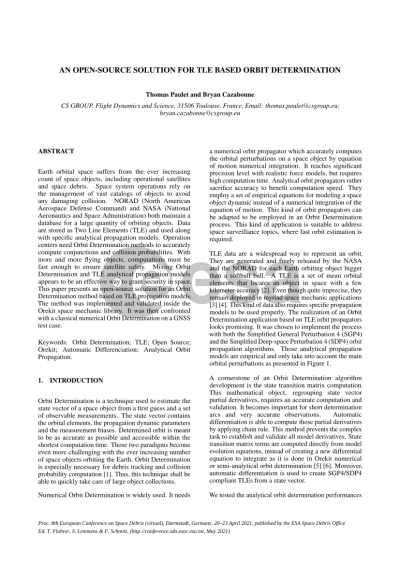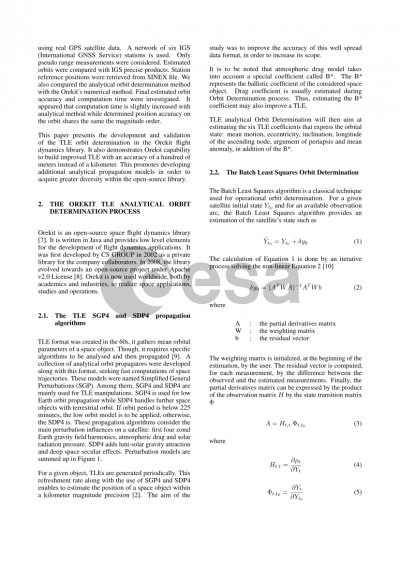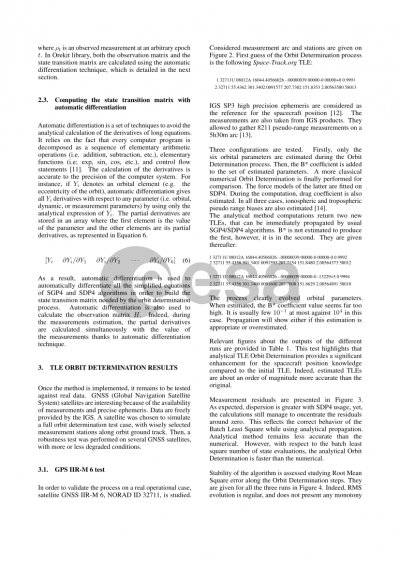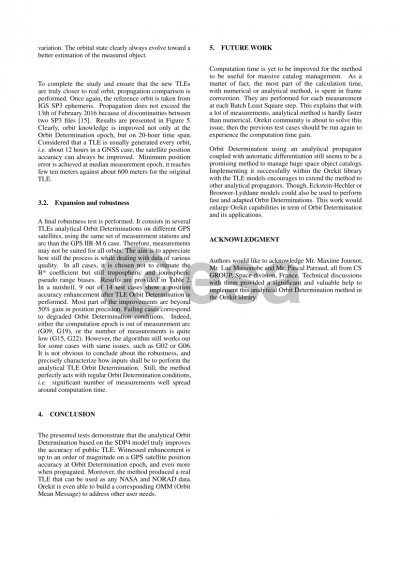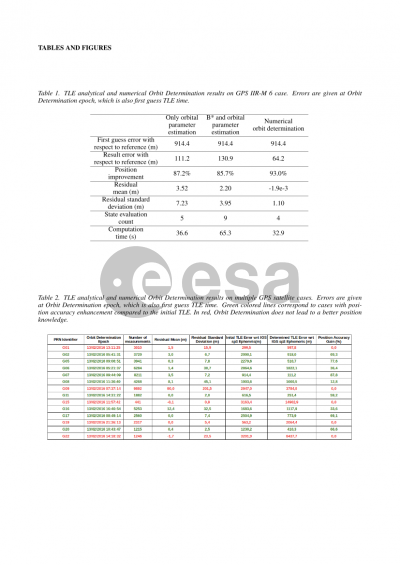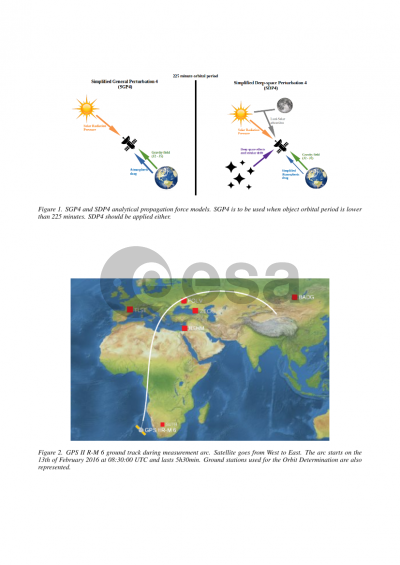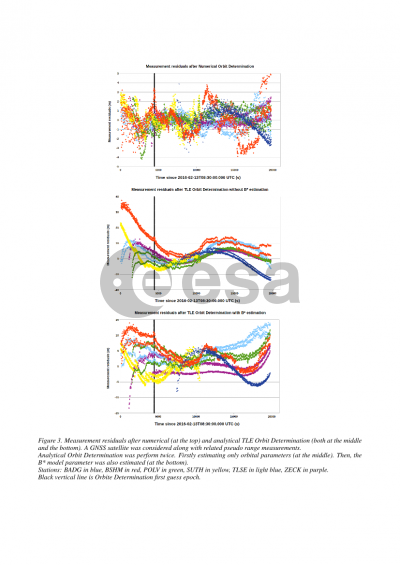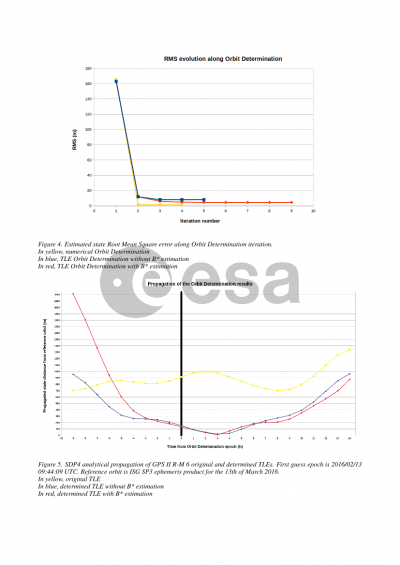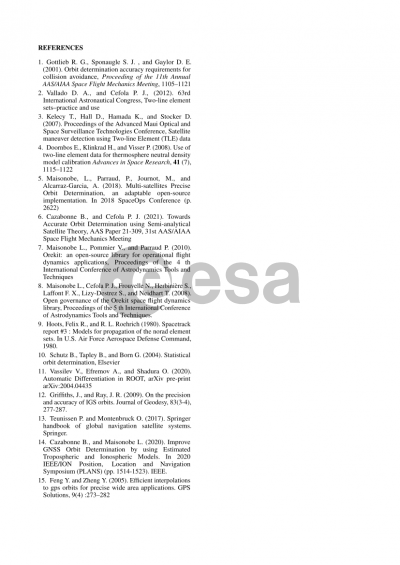# AN OPEN-SOURCE SOLUTION FOR TLE BASED ORBIT DETERMINATION

Thomas Paulet1,Bryan Cazabonne1
CS GROUP1

## Document details

Publishing year2021 PublisherESA Space Debris Office Publishing typeConference Name of conference8th European Conference on Space Debris
Pagesn/a Volume
8
Issue
1
Editors
T. Flohrer, S. Lemmens, F. Schmitz
Download paper## Abstract

Orbit determination is a tool used to estimate the state vector of a space object from a first guess and a collection of observable measurements. The state vector contains the orbital elements, the dynamic parameters and the measurement biases. Determined orbit is meant to be as accurate as possible and accessible with the shortest calculation time. Those two paradigms become even more challenging with the ever increasing number of space object orbiting the Earth. Orbit determination is especially necessary for debris tracking and collision probability computation (R. Gottlieb 2001). Thus, this technique shall be able to quickly take care of a large set of objects.

Numerical orbit determination is widely used. It consists in the use of a numerical orbit propagator which calculates accurately the perturbations on a space object. Therefore, it reaches high precision level with sufficient force models, but it requires significant computation time. On the contrary, analytical orbit propagators sacrifice accuracy to benefit computation speed. They use a set of empirical equations for modeling the dynamic of a space object instead of a numerical integration of the equation of motion. This kind of orbit propagators can be adapted to orbit determination methods in order to obtain a fast determination able to address space surveillance issues.

Two line element (TLE) data are a well spread way to represent an orbit. They are generated and freely released by the NASA and the NORAD for each Earth orbiting object bigger than a softball ball. A TLE gathers mean orbital elements that locate an object with a few kilometer accuracy (Vallado, 2012). Even quite unprecise, they remain deployed in several space mechanic applications (T. Kellecy 2007, E. Doornbos 2008). As specific orbit propagator models must be used to properly propagate a TLE. The realization of an orbit determination based on TLE orbit propagators is interesting. We chose to implement an orbit determination with SGP4 and SDP4 orbit propagation algorithms. Those simple analytical models are empirical and take into account only a 4x4 Earth gravity field, solar radiation pressure and luni-solar attraction.

A cornerstone of the development of an orbit determination algorithm is the computation of state transition matrix. This mathematical object regroups state vector partial derivatives which require precise calculation and validation. Automatic differentiation is able to compute those derivatives applying chain rule. This method prevents the complex task to establish and validate all model derivatives. State transition matrix terms are computed directly from model evolution equations, instead of creating a new differential equation to integrate as in Orekit numerical or semi-analytical orbit determination (B. Cazabonne 2018). Moreover, automatic differentiation is used to create SGP4/SDP4 compliant TLE from a state vector.

In order to test this analytical orbit determination performances, we tested it in reel GPS satellite cases. A network of six IGS station is used. Only pseudo range measurements were considered. Estimated orbits were compared with IGS precise products. Station reference positions were retrieved from SINEX file. We also compared analytical method with Orekit numerical method. Final determined orbit accuracy and computation time were investigated. It appeared that computation time is slightly increased with analytical method while determined position accuracy on the orbit share same the order of magnitude.

This paper presents the development and validation of the TLE orbit determination in Orekit flight dynamic library. It also demonstrates Orekit capability to build improved TLE with an accuracy of a hundred of meters instead of a kilometer. This encourage to develop more analytical propagation models in order to acquire greater diversity of the open-source library.

## Preview